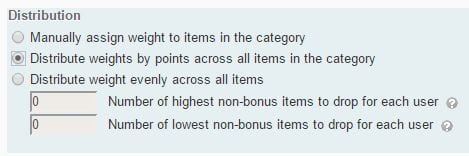It’s not hard to convert a gradebook that doesn’t use categories into a LEARN gradebook with categories. The important thing is to avoid skewing the weights of the individual items.

If the original gradebook is weighted by points:

If the points values are very literal (i.e., a grade item worth 15 points is worth 1/2 as much as a grade item worth 30 points.) then you can simply apply a single setting to each category.For example, my assignments make up 30% of my course.  I will create a category called assignments, giving it a weight of 30%. I would then apply the grade distribution setting of Distribute weights by points across all items in the category”.  When I place my 15 point and 30 point item into that category they have in-category weights of 33.3% and 66.7% which sum to 100% of that category which itself is 30% of the course.

If I added a 3rd item worth 10 points they would then be worth 27.3%, 54.5%, and 18.2% which still sums to 100%.

If the points from your original gradebook do not sum up to 100:

You may want to have your categories weighted exactly the same as your original gradebook.  If your gradebook doesn’t sum out to 100% than you can’t simply add all the items together to find the weight of that category.  You may have to apply some simple math when creating the gradebook.

First the math:
Lets convert the gradebook to sum out of 100.
B / (A / 100)
A = sum of all the grade items.
B = an individual grade item (or category of items).

For example; I have 3 categories of grade items worth 75, 50, and 25.  Lets say that’s the assignments, exams, and quizzes.  A is then 150.  150 / 100 = 1.5.  I would then divide B by 1.5.
75 / 1.5 = 50, 50 / 1.5 = 33.33, and 25 / 1.5 = 16.66.
50 + 33 + 17 = 100

Assignments weight = 50%
Exams weight = 33%
Quizzes weight = 17%